# Reciprocity laws

A number of statements expressing relations between power-residue symbols or norm-residue symbols (cf. Power residue; Norm-residue symbol).

The simplest manifestation of reciprocity laws is the following fact, which was already known to P. Fermat. The only prime divisors of the numbers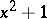areand primes which are terms of the arithmetical series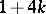. In other words, the identitywhereis a prime, is solvable if and only if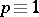. This assertion may be expressed with the aid of the quadratic-residue symbol (Legendre symbol)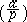as follows: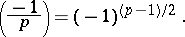In the more general case, the problem of solvability of the congruence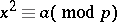(*)

is solved by the Gauss reciprocity law:whereandare different odd primes, and by the following two complements: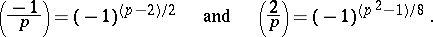These relations for the Legendre symbol show that the prime numbersfor which (*) is solvable for a given non-squareare contained in exactly one-half of the residue classes modulo.

C.F. Gauss recognized the great importance of this reciprocity law and gave several proofs of it, based on completely different concepts . It follows from Gauss' reciprocity law and from its further generalization (the reciprocity law for the Jacobi symbol) that, in particular, the decomposition of a prime numberin a quadratic extension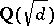of the field of rational numbers(cf. Quadratic field) is determined by the residue class ofmodulo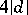.

Gauss' reciprocity law has been generalized to congruences of the form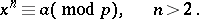However, this involves a transition from the arithmetic of the rational integers to the arithmetic of the integers of an extensionof finite degree of the field of rational numbers. Also, in generalizing the reciprocity law to-th power residues, the extension must be assumed to contain a primitive-th root of unity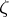. Under this assumption, prime divisorsofwhich are not divisors ofsatisfy the congruencewhere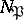is the norm of the divisor, equal to the number of residue classes of the maximal order of this field modulo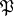. The analogue of the Legendre symbol is defined by the congruenceThe power-residue symbolfor a pair of integersand, analogous to the Jacobi symbol, is defined by the formula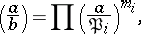ifis the decomposition of the principal divisorinto prime factors andand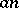are relatively prime.

The reciprocity law forin the field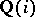was established by Gauss , while that forin the field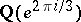was established by G. Eisenstein . E. Kummer  established the general reciprocity law for the power-residue symbol in the field, whereis a prime. Kummer's formula for a regular prime numberhas the formwhere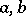are integers in the field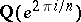,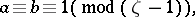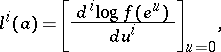and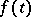is a polynomial of degreesuch that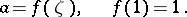The next stage in the study of general reciprocity laws is represented by the work of D. Hilbert , , who cleared up their local aspect. He established, in certain cases, reciprocity laws in the form of a product formula for his norm-residue symbol: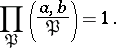He also noted the analogy between this formula and the theorem on residues of algebraic functions — regular pointswith norm-residue symbol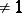correspond to branch points on a Riemann surface.

Further advances in the study of reciprocity laws are due to Ph. Furtwängler , T. Takagi , E. Artin , and H. Hasse . The most general form of the reciprocity law was obtained by I.R. Shafarevich .

Similarly to Gauss' reciprocity law, the general reciprocity law is closely connected with the study of decomposition laws of prime divisorsof a given algebraic number fieldin an algebraic extension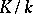with an Abelian Galois group. In particular, class field theory, which offers a solution to this problem, may be based  on Shafarevich's reciprocity law.